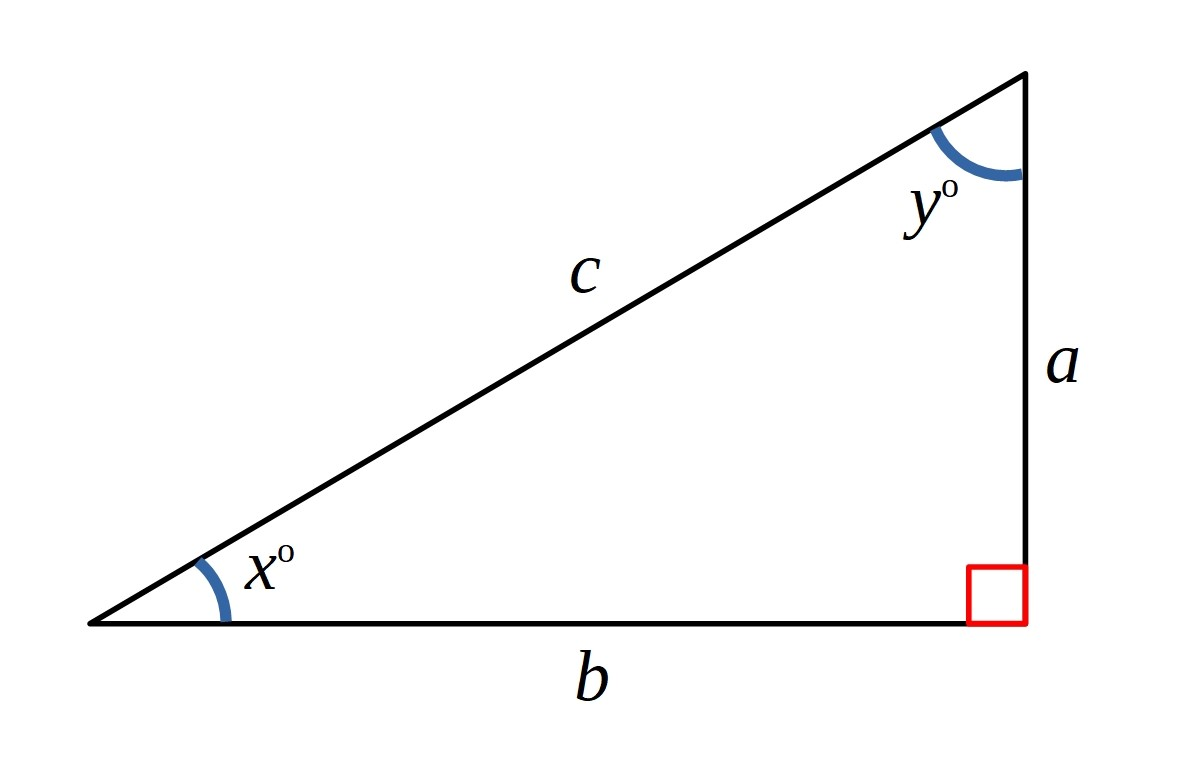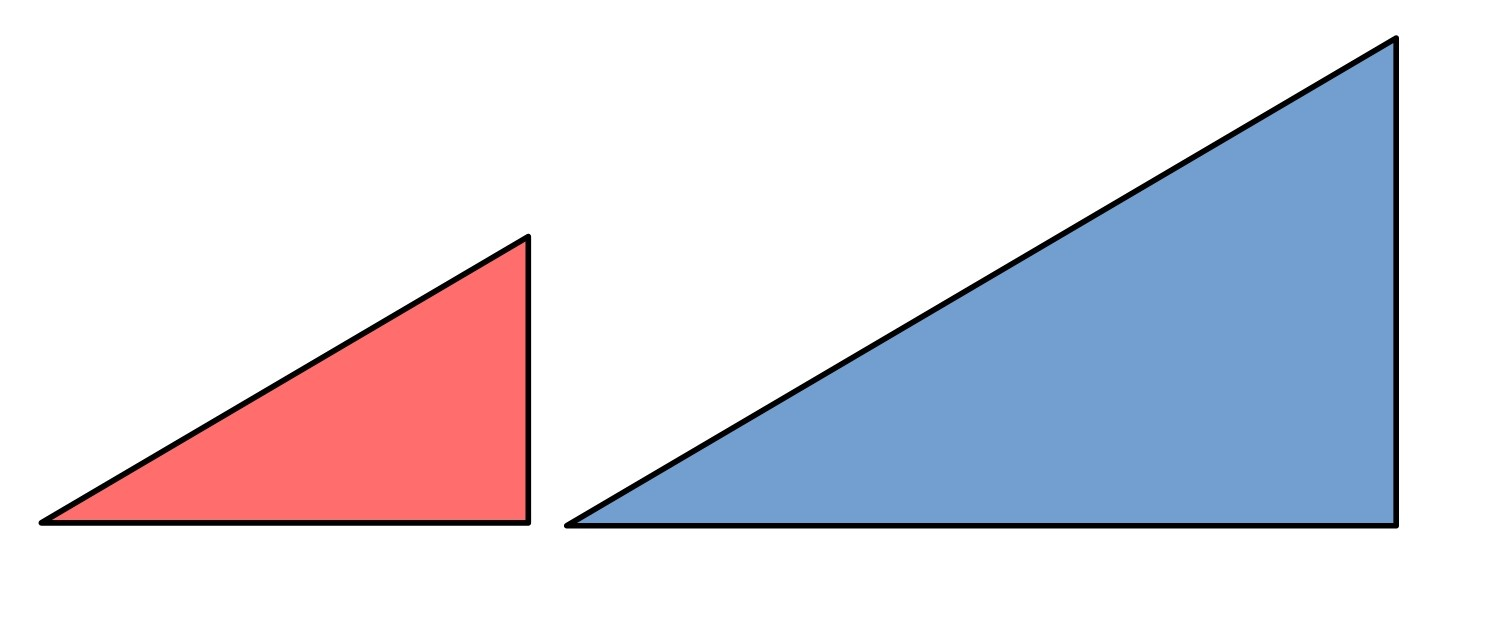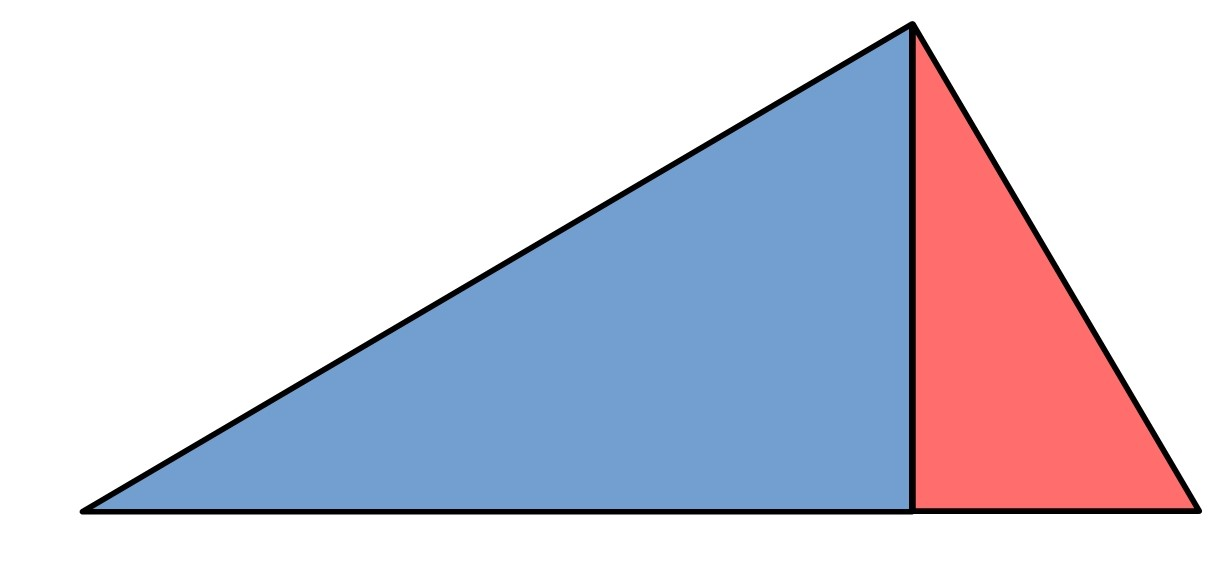#### You may also like### Some(?) of the Parts

A circle touches the lines OA, OB and AB where OA and OB are perpendicular. Show that the diameter of the circle is equal to the perimeter of the triangleA 1 metre cube has one face on the ground and one face against a wall. A 4 metre ladder leans against the wall and just touches the cube. How high is the top of the ladder above the ground?### At a Glance

The area of a regular pentagon looks about twice as a big as the pentangle star drawn within it. Is it?

# Matter of Scale

##### Age 14 to 16Challenge Level

Take any right-angled triangle with side lengths $a, b$ and $c$.  For convenience, label the two acute angles $x^{\circ}$ and $y^{\circ}$.Make two enlargements of the triangle, one by scale factor $a$ and and one by scale factor $b$:What are the lengths of these new triangles?
Draw out a copy of them and indicate what the lengths and the angles are in each.

We can put these two triangles together to make a larger triangle.Find the lengths and angles in this last triangle.
Can you show that this triangle is similar to the original triangle?
What is the scale factor of enlargement between the first and last triangles?

Can you use your results to prove Pythagoras' Theorem?

You might like to explore some more proofs of Pythagoras' Theorem, and a proof of The Converse of Pythagoras' Theorem.

We are very grateful to the Heilbronn Institute for Mathematical Research for their generous support for the development of this resource.